Home > A2C > Chapter 11 > Lesson 11.2.2 > Problem11-63

11-63.
1. Solve each equation. Homework Help ✎

1. log5(2x) = 3

2. log5(x + 1) = −1

3. log(4) − log(x) = 2

4. 2log3(6) + log3(y) = 4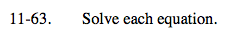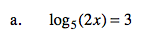Use the definition of a logarithm.

$5^3=2x$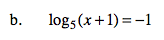See part (a).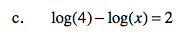Use the properties of logarithms.

$\log\left(\frac{4}{x}\right)=2$

$10^2=\frac{4}{x}$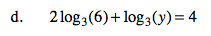$\log_3(6^2y)=4$